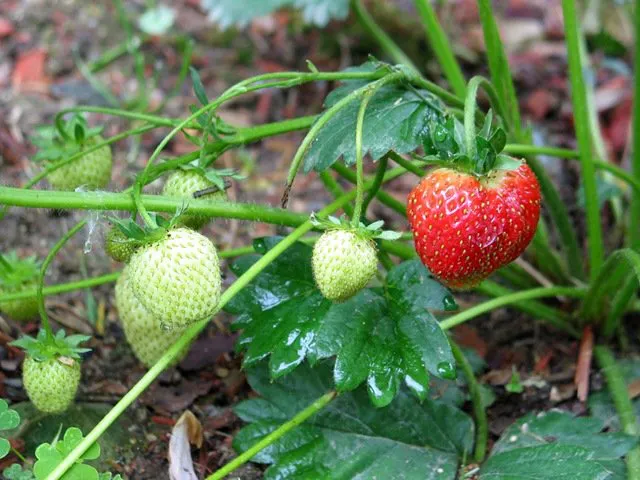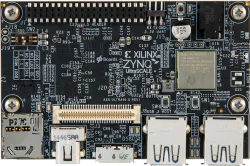# Ripe Fruit Identification

Ripe fruit identification using an Ultra96 board and OpenCV.

IntermediateWork in progress10 hours4,981## Things used in this project

### Hardware componentsAvnet Ultra96
×1
 Web Camera (generic)
×1

### Software apps and online servicesOpenCV

## Code

### Ripe Fruit Identification

Python
```# coding: utf-8

# In:

get_ipython().magic('matplotlib inline')
import cv2
import matplotlib
from matplotlib import colors
from matplotlib import pyplot as plt
import numpy as np
from __future__ import division

# In:

def show(image):
# Figure size in inches
plt.figure(figsize=(15, 15))

# Show image, with nearest neighbour interpolation
plt.imshow(image, interpolation='nearest')

def show_hsv(hsv):
rgb = cv2.cvtColor(hsv, cv2.COLOR_HSV2BGR)
show(rgb)

plt.figure(figsize=(10, 10))

show(img)

# In:

image.shape

# In:

import pandas as pd
m,n,r = image.shape
arr = image.reshape(m*n, -1)
df = pd.DataFrame(arr, columns=['b', 'g', 'r'])
df.describe()

# In:

# Convert from BGR to RGB
image = cv2.cvtColor(image, cv2.COLOR_BGR2RGB)

# Resize to a third of the size
image = cv2.resize(image, None, fx=1/3, fy=1/3)
show(image)

# In:

# Show Red/Green/Blue
images = []
for i in [0, 1, 2]:
colour = image.copy()
if i != 0: colour[:,:,0] = 0
if i != 1: colour[:,:,1] = 0
if i != 2: colour[:,:,2] = 0
images.append(colour)

show(np.vstack(images))

# In:

def show_rgb_hist(image):
colours = ('r','g','b')
for i, c in enumerate(colours):
plt.figure(figsize=(20, 4))
histr = cv2.calcHist([image], [i], None, , [0, 256])
#         plt.plot(histr, color=c, lw=2)

if c == 'r': colours = [((i/256, 0, 0)) for i in range(0, 256)]
if c == 'g': colours = [((0, i/256, 0)) for i in range(0, 256)]
if c == 'b': colours = [((0, 0, i/256)) for i in range(0, 256)]

plt.bar(range(0, 256), histr, color=colours, edgecolor=colours, width=1)
#         plt.xlim([0, 256])

plt.show()

show_rgb_hist(image)

# In:

# Convert from RGB to HSV
hsv = cv2.cvtColor(image, cv2.COLOR_RGB2HSV)

images = []
for i in [0, 1, 2]:
colour = hsv.copy()
if i != 0: colour[:,:,0] = 0
if i != 1: colour[:,:,1] = 255
if i != 2: colour[:,:,2] = 255
images.append(colour)

hsv_stack = np.vstack(images)
rgb_stack = cv2.cvtColor(hsv_stack, cv2.COLOR_HSV2RGB)
show(rgb_stack)

# In:

matplotlib.rcParams.update({'font.size': 16})

def show_hsv_hist(image):
# Hue
plt.figure(figsize=(20, 3))
histr = cv2.calcHist([image], , None, , [0, 180])
plt.xlim([0, 180])
colours = [colors.hsv_to_rgb((i/180, 1, 0.9)) for i in range(0, 180)]
plt.bar(range(0, 180), histr, color=colours, edgecolor=colours, width=1)
plt.title('Hue')

# Saturation
plt.figure(figsize=(20, 3))
histr = cv2.calcHist([image], , None, , [0, 256])
plt.xlim([0, 256])

colours = [colors.hsv_to_rgb((0, i/256, 1)) for i in range(0, 256)]
plt.bar(range(0, 256), histr, color=colours, edgecolor=colours, width=1)
plt.title('Saturation')

# Value
plt.figure(figsize=(20, 3))
histr = cv2.calcHist([image], , None, , [0, 256])
plt.xlim([0, 256])

colours = [colors.hsv_to_rgb((0, 1, i/256)) for i in range(0, 256)]
plt.bar(range(0, 256), histr, color=colours, edgecolor=colours, width=1)
plt.title('Value')

#show_hsv_hist(hsv)

# In:

# Blur image slightly
image_blur = cv2.GaussianBlur(image, (7, 7), 0)
show(image_blur)

# In:

image_blur_hsv = cv2.cvtColor(image_blur, cv2.COLOR_RGB2HSV)

# 0-10 hue
min_red = np.array([100, 100, 0])
max_red = np.array([55, 10, 51])
image_red1 = cv2.inRange(image_blur_hsv, min_red, max_red)

# 170-180 hue
min_red2 = np.array([170, 100, 60])
max_red2 = np.array([180, 256, 256])
image_red2 = cv2.inRange(image_blur_hsv, min_red2, max_red2)

image_red = image_red1 + image_red2

# In:

image_red

# In:

# Clean up
kernel = cv2.getStructuringElement(cv2.MORPH_ELLIPSE, (15, 15))

# image_red_eroded = cv2.morphologyEx(image_red, cv2.MORPH_ERODE, kernel)

# image_red_dilated = cv2.morphologyEx(image_red, cv2.MORPH_DILATE, kernel)

# image_red_opened = cv2.morphologyEx(image_red, cv2.MORPH_OPEN, kernel)

# Fill small gaps
image_red_closed = cv2.morphologyEx(image_red, cv2.MORPH_CLOSE, kernel)

# Remove specks
image_red_closed_then_opened = cv2.morphologyEx(image_red_closed, cv2.MORPH_OPEN, kernel)

# In:

def find_biggest_contour(image):

# Copy to prevent modification
image = image.copy()
img, contours, hierarchy = cv2.findContours(image, cv2.RETR_LIST, cv2.CHAIN_APPROX_SIMPLE)
#print len(contours)

# Isolate largest contour
contour_sizes = [(cv2.contourArea(contour), contour) for contour in contours]
biggest_contour = max(contour_sizes, key=lambda x: x)

# In:

# In:

# Centre of mass
centre_of_mass = int(moments['m10'] / moments['m00']), int(moments['m01'] / moments['m00'])
image_with_com = image.copy()
cv2.circle(image_with_com, centre_of_mass, 10, (0, 255, 0), -1)
show(image_with_com)

# Bounding ellipse
image_with_ellipse = image.copy()
ellipse = cv2.fitEllipse(big_contour)
cv2.ellipse(image_with_ellipse, ellipse, (0,255,0), 2)
show(image_with_ellipse)
```

## Credits

### Md. Khairul Alam

43 projects • 401 followers
Engineer, developer, maker & hacker. Currently working as a faculty at the University of Asia Pacific, Dhaka, Bangladesh.